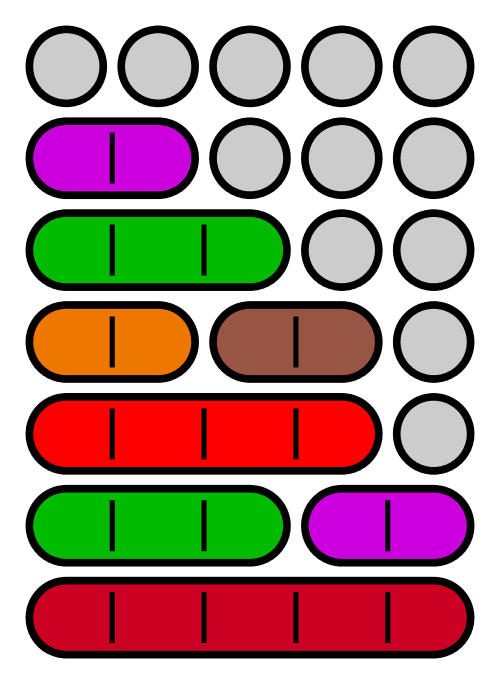# That's the only numbers I know

Probability Level 2The number of seven digit integers, with the sum of digits equal to 10 and formed by using the digits 1,2 and 3 only is,